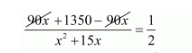# A train covers a distance of 90 km at a uniform speed.

Question:

A train covers a distance of 90 km at a uniform speed. Had the speed been 15 km/hour more, it would have taken 30 minutes less for a journey. Find the original speed of the train.

Solution:

Let the original speed of train be $x \mathrm{~km} / \mathrm{hr}$. Then,

Increased speed of the train $=(x+15) \mathrm{km} / \mathrm{hr}$

Time taken by the train under usual speed to cover $90 \mathrm{~km}=\frac{90}{x} \mathrm{hr}$

Time taken by the train under increased speed to cover $90 \mathrm{~km}=\frac{90}{(x+15)} \mathrm{hr}$

Therefore,

$\frac{90}{x}-\frac{90}{(x+15)}=\frac{30}{60}$

$\frac{\{90(x+15)-90 x\}}{x(x+15)}=\frac{1}{2}$

$\frac{90 x+1350-90 x}{x^{2}+15 x}=\frac{1}{2}$$2700=x^{2}+15 x$

$x^{2}+15 x-2700=0$

$x^{2}+15 x-2700=0$

$x^{2}-45 x+60 x-2700=0$

$x(x-45)+60(x-45)=0$

$(x-45)(x+60)=0$

So, either

$(x-45)=0$

$x=45$

Or

$(x+60)=0$

$x=-60$

But, the speed of the train can never be negative.

Hence, the original speed of train is $x=45 \mathrm{~km} / \mathrm{hr}$